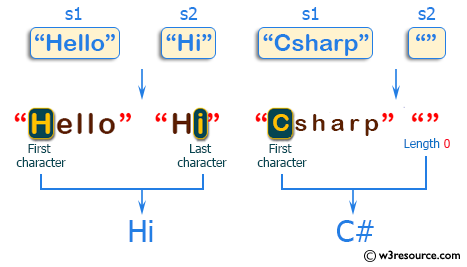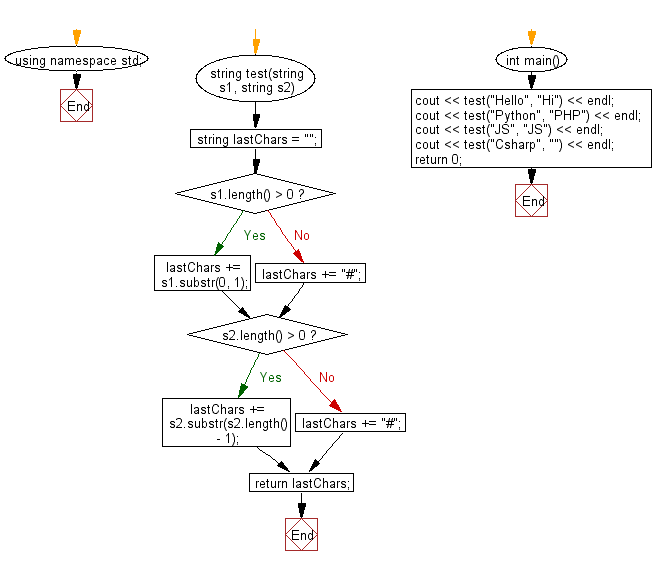﻿ C++ : Combining first and last characters from two strings# C++ Exercises: Create a new string taking the first character from a given string and the last character from another given string

## C++ Basic Algorithm: Exercise-72 with Solution

Write a C++ program to create a new string taking the first character from a given string and the last character from another given string. If the length of any given string is 0, use '#' as its missing character.

Sample Solution:

C++ Code :

``````#include <iostream>

using namespace std;

string test(string s1, string s2)
{
string lastChars = "";

if (s1.length() > 0)
{
lastChars += s1.substr(0, 1);
}
else
{
lastChars += "#";
}

if (s2.length() > 0)
{
lastChars += s2.substr(s2.length() - 1);
}
else
{
lastChars += "#";
}

return lastChars;
}

int main()
{
cout << test("Hello", "Hi") << endl;
cout << test("Python", "PHP") << endl;
cout << test("JS", "JS") << endl;
cout << test("Csharp", "") << endl;
return 0;
}
``````

Sample Output:

```Hi
PP
JS
C#
```

Pictorial Presentation:Flowchart:C++ Code Editor:

Contribute your code and comments through Disqus.

What is the difficulty level of this exercise?

﻿